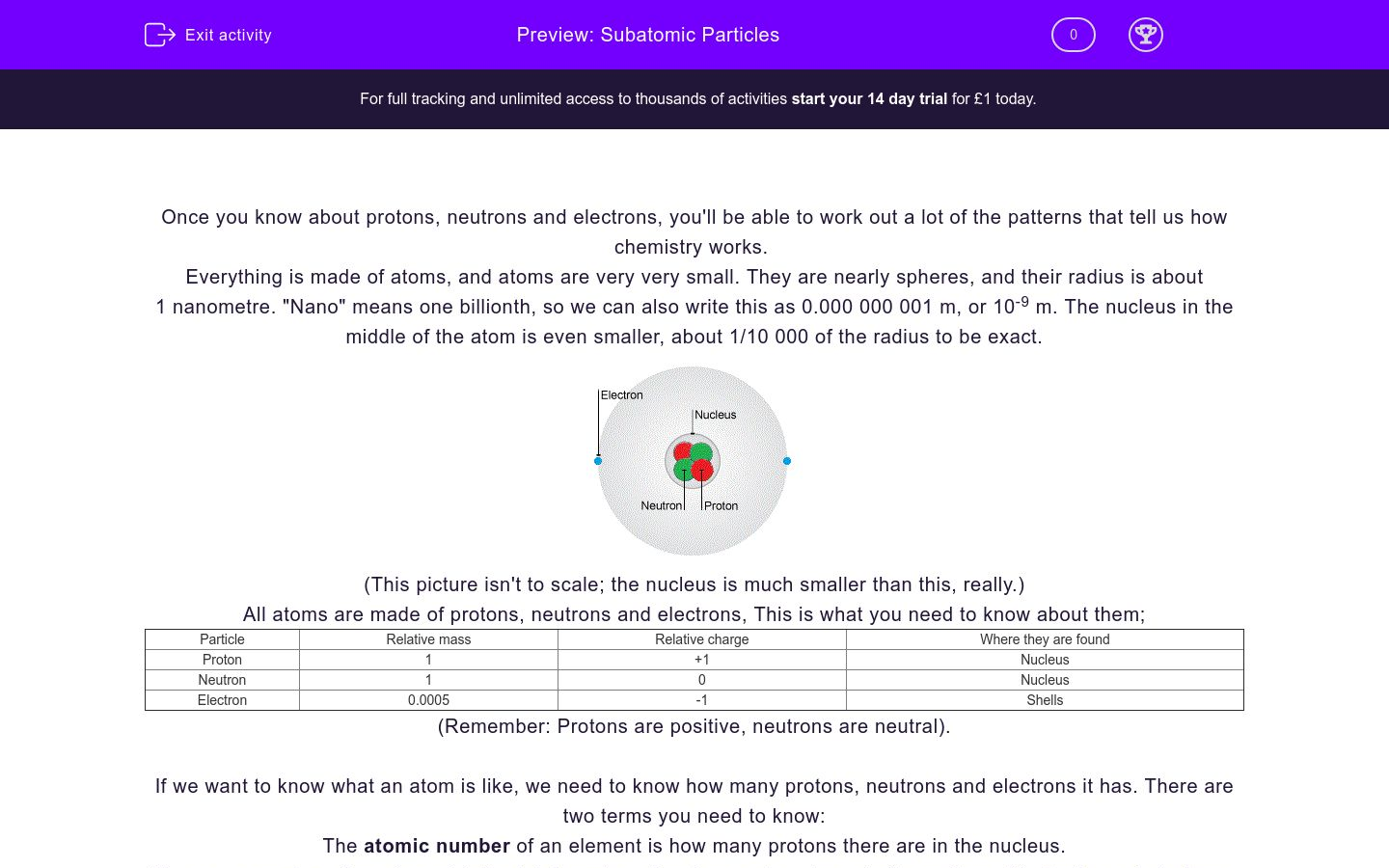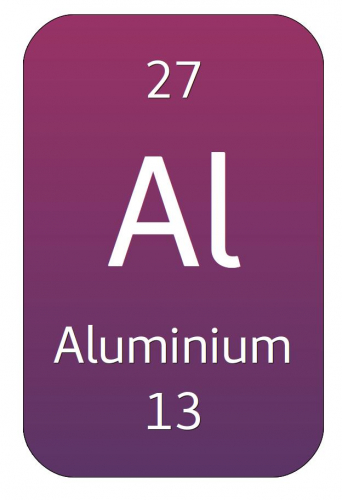# Subatomic Particles

In this worksheet, students will learn about protons, neutrons and electrons, and the way they join together to make atoms.Key stage:  KS 4

GCSE Boards:   AQA, AQA Trilogy, AQA Synergy, OCR 21st Century, OCR Gateway, Pearson Edexcel

Difficulty level:### QUESTION 1 of 10

Once you know about protons, neutrons and electrons, you'll be able to work out a lot of the patterns that tell us how chemistry works.

Everything is made of atoms, and atoms are very very small. They are nearly spheres, and their radius is about 1 nanometre. "Nano" means one billionth, so we can also write this as 0.000 000 001 m, or 10-9 m. The nucleus in the middle of the atom is even smaller, about 1/10 000 of the radius to be exact.(This picture isn't to scale; the nucleus is much smaller than this, really.)

All atoms are made of protons, neutrons and electrons, This is what you need to know about them;

 Particle Relative mass Relative charge Where they are found Proton 1 +1 Nucleus Neutron 1 0 Nucleus Electron 0.0005 -1 Shells

(Remember: Protons are positive, neutrons are neutral).

If we want to know what an atom is like, we need to know how many protons, neutrons and electrons it has. There are two terms you need to know:

The atomic number of an element is how many protons there are in the nucleus.

The mass number of an element is the total number of protons and neutrons in the nucleus. We don't count electrons, because they are much lighter.

When you look up an element in the periodic table, it will tell you the atomic number and mass number, like this:Different periodic tables put the numbers in different places, so remember that the larger number is the mass number, and the smaller number is the atomic number. It's also a good idea to get a copy of the version of the periodic table your exam board uses, so you can get used to it.

To work out the numbers of protons, neutrons and electrons, follow these rules:

The number of protons is the same as the atomic number.

So sodium has 11 protons

The number of neutrons is the difference between the mass number and the atomic number.

So the number of neutrons in sodium = 23 - 11 = 12 neutrons

The number of electrons in an atom is the same as the number of protons.

So sodium atoms have 11 electrons

These questions can seem a bit scary to start with, but all you have to do is adding and subtracting. Once you've done some for yourself, it becomes easy to talk about atomic numbers and mass numbers.

Which of these is the radius of an atom? Tick all the right answers.

A nanometre

0.000 000 001 m

1/10 000 m

The size of the nucleus

Which of these describe a proton? Tick all the ones that apply.

Charge = +1

Charge = -1

Mass = 1

Mass = 0

Which of these statements correctly describes an electron?

charge = +1, mass = 0.0005, in the nucleus

charge = -1, mass = 1, in the nucleus

charge = -1, mass = 0.0005, in shells

Which particles are found in the nucleus? Tick all the right answers.

Croutons

Electrons

Neutrons

Protons

Match these phrases with their definitions.

## Column B

Atomic number
Total number of protons and neutrons in the nucleu...
Mass number
Central part of the atom, containing protons and n...
Nucleus
Number of protons in the nucleus

The atomic number of fluorine is 9, and the mass number is 19. How many protons are there in the fluorine nucleus?

1

9

10

19

The atomic number of fluorine is 9, and the mass number is 19. How many neutrons are there in a nucleus of fluorine?

1

9

10

19

The atomic number of fluorine is 9, and the mass number is 19. How many electrons does a fluorine atom have?

The periodic table box for aluminium looks like this:Match up these half sentences about aluminium.

## Column B

The atomic number is
13, because that's the smaller number
The mass number is
27, because that's the larger number
The number of neutrons is
14, because that's the difference between the mass...

The box in the periodic table for lithium looks like this:Use this information to complete the description of the lithium atom.

## Column B

The atomic number is
13, because that's the smaller number
The mass number is
27, because that's the larger number
The number of neutrons is
14, because that's the difference between the mass...
• Question 1

Which of these is the radius of an atom? Tick all the right answers.

A nanometre
0.000 000 001 m
EDDIE SAYS
Sometimes, people talk about "nanotechnology" in the news. This means technology with single atoms, because atoms are about a nanometre radius.
• Question 2

Which of these describe a proton? Tick all the ones that apply.

Charge = +1
Mass = 1
EDDIE SAYS
Remember, protons are positive. Protons and neutrons both have a relative mass of 1.
• Question 3

Which of these statements correctly describes an electron?

charge = -1, mass = 0.0005, in shells
EDDIE SAYS
Have another look at the table in the introduction if you're not sure. Electrons are negatively charged, very light and are found in shells around the nucleus.
• Question 4

Which particles are found in the nucleus? Tick all the right answers.

Neutrons
Protons
EDDIE SAYS
Check the picture of the atomic structure if you're not sure about this. It's one of the diagrams you really need to know by heart.
• Question 5

Match these phrases with their definitions.

## Column B

Atomic number
Number of protons in the nucleus
Mass number
Total number of protons and neutr...
Nucleus
Central part of the atom, contain...
EDDIE SAYS
You might be asked about these definitions in an exam, so take care to get them the right way round. As you do some calculations, it will help you remember which way round they go.
• Question 6

The atomic number of fluorine is 9, and the mass number is 19. How many protons are there in the fluorine nucleus?

9
EDDIE SAYS
The atomic number is the number of protons, so you don't need to do any addition or subtraction.
• Question 7

The atomic number of fluorine is 9, and the mass number is 19. How many neutrons are there in a nucleus of fluorine?

10
EDDIE SAYS
To get the number of neutrons, find the difference between the mass number (that's protons + neutrons) and the atomic number (that's protons only). So 19 - 9 = 10.
• Question 8

The atomic number of fluorine is 9, and the mass number is 19. How many electrons does a fluorine atom have?

9
EDDIE SAYS
For an atom, there are equal numbers of protons and electrons, so atoms have no overall charge. Atoms can gain or lose electrons (then they turn into ions)... which is what happens in a lot of chemical reactions.
• Question 9

The periodic table box for aluminium looks like this:Match up these half sentences about aluminium.

## Column B

The atomic number is
13, because that's the smaller nu...
The mass number is
27, because that's the larger num...
The number of neutrons is
14, because that's the difference...
EDDIE SAYS
Most printed periodic tables tell you which number is the atomic number and which is the mass number. It's much better to think "mass number is larger, atomic number is smaller", because then it doesn't matter what the printed layout is.
• Question 10

The box in the periodic table for lithium looks like this:Use this information to complete the description of the lithium atom.

EDDIE SAYS
There's a lot going on here, so don't worry if you found it harder. There are two numbers for each element- the larger one is the mass number, and the smaller one is the atomic number. The atomic number gives you both the protons and the electrons. To get the neutron number, subtract atomic number from mass number.
---- OR ----

Sign up for a £1 trial so you can track and measure your child's progress on this activity.

### What is EdPlace?

We're your National Curriculum aligned online education content provider helping each child succeed in English, maths and science from year 1 to GCSE. With an EdPlace account you’ll be able to track and measure progress, helping each child achieve their best. We build confidence and attainment by personalising each child’s learning at a level that suits them.

Get started